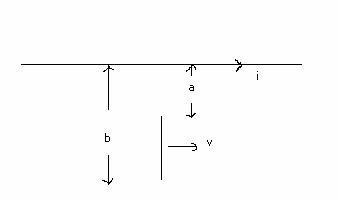# Induced EMF on a moving rod next to a line of current.

## Homework Statement

I'm currently reviewing some of the old E&M material that I haven't seen in a while and have gotten stuck on this problem. I'm sure I'm just making a simple mistake but I can't seem to make any headway.

Here's the problem: Figure 35-32 shows a copper rod moving with velocity v parallel to a long straight wire carrying a current i. Calculate the induced emf in the rod, assuming v = 5.0m/s, i = 100A, a = 1cm and b = 20cm. Answer: 3.0X10-4V

## The Attempt at a Solution

The magnetic field of the wire a distance y away is $$B = \frac{\mu _{0}i}{2\pi y}$$. Thus the total magnetic field across the length of the rod is $$B = \frac{\mu_{0}i}{2\pi}\int_{a}^{b}\frac{dy}{y} = \frac{\mu_{0}i}{2\pi}ln\frac{b}{a}$$. Now the induced emf is $$\varepsilon = -\frac{d\Phi _{B}}{dt}=-B\frac{dA}{dt}=-B(b-a)\frac{dx}{dt}=-B(b-a)v$$. Then the total equation is $$\varepsilon = \frac{-\mu_{0}iv}{2\pi}(b-a)ln\frac{b}{a}$$, the only problem being that this is apparently not the correct answer.tiny-tim
Homework Helper
hi grindfreak!Now the induced emf is $$\varepsilon = -\frac{d\Phi _{B}}{dt}=-B\frac{dA}{dt}=-B(b-a)\frac{dx}{dt}=-B(b-a)v$$.

i don't understand this at allyes, the area swept out in time t is A = (b-a)vt

but the magnetic flux through that area is ∫ab B(y)vt dy,

ie your previous integral times vt

how did you get that (b-a) factor?
Thus the total magnetic field across the length of the rod is $$B = \frac{\mu_{0}i}{2\pi}\int_{a}^{b}\frac{dy}{y} = \frac{\mu_{0}i}{2\pi}ln\frac{b}{a}$$.

whatever is "total magnetic field"??if you mean the flux, then say so, and don't call it "B" !

your strange nomenclature seems to have confused you

Yes I think your first integral is probably the right way to go with it, the b-a factor is the length of the rod, so that (b-a)*x is the area swept out by the rod in time t. As for total magnetic field, I really should have said magnetic field on the rod by the line of current (sorry if this sounds a bit poorly worded, I've been working all day).

tiny-tim
get some sleep :zzz:, and it'll be clearer in the morning